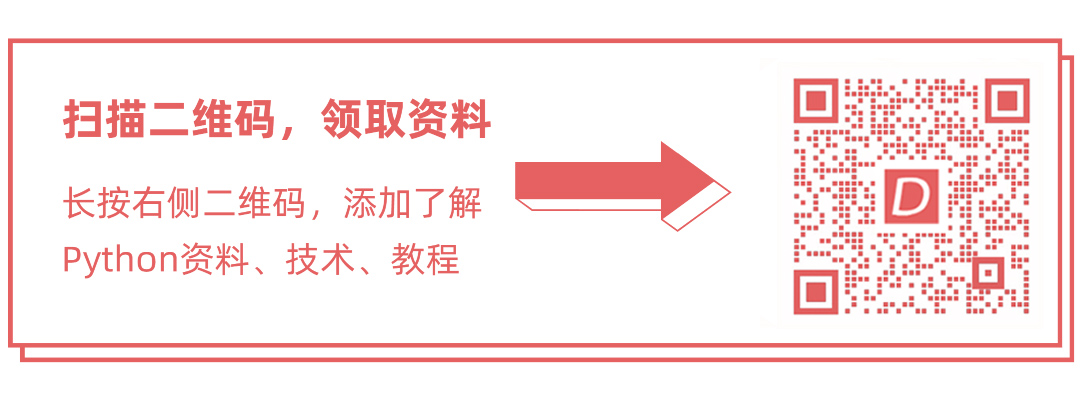### 史上最全Python快速入门教程

Python是面向对象，高级语言，解释，动态和多用途编程语言。Python易于学习，而且功能强大，功能多样的脚本语言使其对应用程序开发具有吸引力。

Python的语法和动态类型具有其解释性质，使其成为许多领域的脚本编写和快速应用程序开发的理想语言。

Python支持多种编程模式，包括面向对象编程，命令式和函数式编程或过程式编程。

Python使开发和调试快速，因为在python开发中没有包含编译步骤，并且编辑 <-> 测试 <-> 调试循环使用代码开发效率非常高。Python是一种高级，解释，交互和面向对象的脚本语言。 Python被设计为高度可读性。 它使用英语关键字，而其他语言使用标点符号。它的语法结构比其他语言少。

• Python是解释型语言 – Python代码在解释器中运行时处理，执行前不需要编译程序。 这与PERL和PHP类似。
• Python是交动的 – 在Python提示符下面直接和解释器进行交互来编写程序。
• Python是面向对象的 – Python支持面向对象的风格或编程技术，将代码封装在对象内。
• Python是一门初学者的语言 – Python是初学者程序员的伟大语言，并支持从简单的文本处理到WWW浏览器到游戏的各种应用程序的开发。

## 第一节 Python 可以用来开发什么？

Python作为一个整体可以用于任何软件开发领域。下面来看看Python可以应用在哪些领域的开发。如下所列 –

## 1.基于控制台的应用程序

Python可用于开发基于控制台的应用程序。 例如：IPython。

## 2.基于音频或视频的应用程序

Python在多媒体部分开发，证明是非常方便的。 一些成功的应用是：TimPlayer，cplay等。

## 4.Web应用程序

Python也可以用于开发基于Web的应用程序。 一些重要的开发案例是：PythonWikiEngines，Pocoo，PythonBlogSoftware等，如国内的成功应用案例有：豆瓣，知乎等。

## 5.企业级应用

Python可用于创建可在企业或组织中使用的应用程序。一些实时应用程序是：OpenErp，Tryton，Picalo等。

## 第二节 Python安装和环境配置

Python 3适用于Windows，Mac OS和大多数Linux操作系统。即使Python 2目前可用于许多其他操作系统，有部分系统Python 3还没有提供支持或者支持了但被它们在系统上删除了，只保留旧的Python 2版本。

## 第三节 Python命令行参数

Python提供了一个getopt模块，用于解析命令行选项和参数。

``````\$ python test.py arg1 arg2 arg3Shell
``````
• 1

Python sys模块通过sys.argv提供对任何命令行参数的访问。主要有两个参数变量 –

• sys.argv是命令行参数的列表。
• len(sys.argv)是命令行参数的数量。

## 示例

``````#!/usr/bin/python3import sysprint ('Number of arguments:', len(sys.argv), 'arguments.')print ('Argument List:', str(sys.argv))Python
``````
• 1

``````F:\>python F:\worksp\python\command_line_arguments.pyNumber of arguments: 1 arguments.Argument List: ['F:\\worksp\\python\\command_line_arguments.py']F:\>python F:\worksp\python\command_line_arguments.py arg1 arg2 arg3 arg4Number of arguments: 5 arguments.Argument List: ['F:\\worksp\\python\\command_line_arguments.py', 'arg1', 'arg2', 'arg3', 'arg4']F:\>Python
``````
• 1

## 解析命令行参数

Python提供了一个getopt模块，可用于解析命令行选项和参数。该模块提供了两个功能和异常，以启用命令行参数解析。

getopt.getopt方法

``````getopt.getopt(args, options, [long_options])Python
``````
• 1

getopt.GetoptError异常

## 示例

``````usage: file.py -i <inputfile> -o <outputfile>Shell
``````
• 1

``````#!/usr/bin/python3import sys, getoptdef main(argv):   inputfile = ''   outputfile = ''   try:      opts, args = getopt.getopt(argv,"hi:o:",["ifile=","ofile="])   except getopt.GetoptError:      print ('GetoptError, usage: command_line_usage.py -i <inputfile> -o <outputfile>')      sys.exit(2)   for opt, arg in opts:      if opt == '-h':         print ('usage: command_line_usage.py -i <inputfile> -o <outputfile>')         sys.exit()      elif opt in ("-i", "--ifile"):         inputfile = arg      elif opt in ("-o", "--ofile"):         outputfile = arg   print ('Input file is "', inputfile)   print ('Output file is "', outputfile)if __name__ == "__main__":   main(sys.argv[1:])Python
``````
• 1

``````F:\worksp\python>python command_line_usage.py -husage: command_line_usage.py -i <inputfile> -o <outputfile>F:\worksp\python>python command_line_usage.py -i inputfile.txt -oGetoptError, usage: command_line_usage.py -i <inputfile> -o <outputfile>F:\worksp\python>python command_line_usage.py -i inputfile.txt -o outputfile.txtInput file is " inputfile.txtOutput file is " outputfile.txtF:\worksp\python>Shell
``````
• 1

## 第四节 Python变量类型## 将值分配给变量

=运算符左侧的操作数是变量的名称，而=运算符右侧的操作数是将在存储在变量中的值。 例如 –

``````#!/usr/bin/python3counter = 100          # 一个整型数miles   = 999.99       # 一个浮点数name    = "Maxsu"       # 一个字符串site_url  = "http://www.yiibai.com" # 一个字符串print (counter)print (miles)print (name)print (site_url)Python
``````
• 1

``````100999.99 Maxsuhttp://www.yiibai.comShell
``````
• 1

## 多重赋值

Python允许同时为多个变量分配单个值。

``````a = b = c = 1Python
``````
• 1

``````a, b, c = 10, 20, "maxsu"Python
``````
• 1

## 标准数据类型

Python有五种标准数据类型 –

• 1.数字
• 2.字符串
• 3.列表
• 4.元组
• 5.字典

## 1.Python数字

``````var1 = 10var2 = 20Python
``````
• 1

``````del var1[,var2[,var3[....,varN]]]]Python
``````
• 1

``````del vardel var_a, var_bPython
``````
• 1

Python支持三种不同的数值类型 –

• int(有符号整数)
• float(浮点实值)
• complex(复数)

Python3中的所有整数都表示为长整数。 因此，长整数没有单独的数字类型。

## 例子

int float complex 10 0.0 3.14j 100 15.20 45.j -786 -21.9 9.322e-36j 080 32.3+e18 .876j -0490 -90. -.6545+0J -0x260 -32.54e100 3e+26J 0x69 70.2-E12 4.53e-7j

## 2.Python字符串

Python中的字符串被标识为在引号中表示的连续字符集。Python允许双引号或双引号。 可以使用片段运算符([]和[:])来获取字符串的子集(子字符串)，其索引从字符串开始处的索引0开始，并且以-1表示字符串中的最后一个字符。

``````#!/usr/bin/python3#coding=utf-8# save file: variable_types_str1.pystr = 'yiibai.com'print ('str = ', str)          # Prints complete stringprint ('str = ',str)       # Prints first character of the stringprint ('str[2:5] = ',str[2:5])     # Prints characters starting from 3rd to 5thprint ('str[2:] = ',str[2:])      # Prints string starting from 3rd characterprint ('str[-1] = ',str[-1])      # 最后一个字符，结果为：'!'print ('str * 2 = ',str * 2)      # Prints string two timesprint ('str + "TEST" = ',str + "TEST") # Prints concatenated stringPython
``````
• 1

``````F:\worksp\python>python variable_types_str1.pystr =  yiibai.comstr =  ystr[2:5] =  ibastr[2:] =  ibai.comstr[-1] =  mstr * 2 =  yiibai.comyiibai.comstr + "TEST" =  yiibai.comTESTF:\worksp\python>Shell
``````
• 1

## 2.Python列表

``````#!/usr/bin/python3#coding=utf-8# save file: variable_types_str1.pylist = [ 'yes', 'no', 786 , 2.23, 'minsu', 70.2 ]tinylist = [100, 'maxsu']print ('list = ', list)          # Prints complete listprint ('list = ',list)       # Prints first element of the listprint ('list[1:3] = ',list[1:3])     # Prints elements starting from 2nd till 3rd print ('list[2:] = ',list[2:])      # Prints elements starting from 3rd elementprint ('list[-3:-1] = ',list[-3:-1])    print ('tinylist * 2 = ',tinylist * 2)  # Prints list two timesprint ('list + tinylist = ', list + tinylist) # Prints concatenated listsPython
``````
• 1

``````F:\worksp\python>python variable_types_list.pylist =  ['yes', 'no', 786, 2.23, 'minsu', 70.2]list =  yeslist[1:3] =  ['no', 786]list[2:] =  [786, 2.23, 'minsu', 70.2]list[-3:-1] =  [2.23, 'minsu']tinylist * 2 =  [100, 'maxsu', 100, 'maxsu']list + tinylist =  ['yes', 'no', 786, 2.23, 'minsu', 70.2, 100, 'maxsu']F:\worksp\python>Shell
``````
• 1

## 3.Python元组

``````#!/usr/bin/python3#coding=utf-8# save file : variable_types_tuple.pytuple = ( 'maxsu', 786 , 2.23, 'yiibai', 70.2  )tinytuple = (999.0, 'maxsu')# tuple = 'new item value' 不能这样赋值print ('tuple = ', tuple)           # Prints complete tupleprint ('tuple = ', tuple)        # Prints first element of the tupleprint ('tuple[1:3] = ', tuple[1:3])      # Prints elements starting from 2nd till 3rd print ('tuple[-3:-1] = ', tuple[-3:-1])       # 输出结果是什么？print ('tuple[2:] = ', tuple[2:])       # Prints elements starting from 3rd elementprint ('tinytuple * 2 = ',tinytuple * 2)   # Prints tuple two timesprint ('tuple + tinytuple = ', tuple + tinytuple) # Prints concatenated tuplePython
``````
• 1

``````F:\worksp\python>python variable_types_tuple.pytuple =  ('maxsu', 786, 2.23, 'yiibai', 70.2)tuple =  maxsutuple[1:3] =  (786, 2.23)tuple[-3:-1] =  (2.23, 'yiibai')tuple[2:] =  (2.23, 'yiibai', 70.2)tinytuple * 2 =  (999.0, 'maxsu', 999.0, 'maxsu')tuple + tinytuple =  ('maxsu', 786, 2.23, 'yiibai', 70.2, 999.0, 'maxsu')F:\worksp\python>Shell
``````
• 1

``````#!/usr/bin/python3tuple = ( 'abcd', 786 , 2.23, 'john', 70.2  )list = [ 'abcd', 786 , 2.23, 'john', 70.2  ]tuple = 1000    # 无法更新值，程序出错list = 1000     # 有效的更新，合法Python
``````
• 1

## Python字典

Python的字典是一种哈希表类型。它们像Perl中发现的关联数组或散列一样工作，由键值对组成。字典键几乎可以是任何Python数据类型，但通常为了方便使用数字或字符串。另一方面，值可以是任意任意的Python对象。

``````#!/usr/bin/python3#coding=utf-8# save file : variable_types_dict.pydict = {}dict['one'] = "This is one"dict     = "This is my"tinydict = {'name': 'maxsu', 'code' : 1024, 'dept':'IT Dev'}print ("dict['one'] = ", dict['one'])       # Prints value for 'one' keyprint ('dict = ', dict)           # Prints value for 2 keyprint ('tinydict = ', tinydict)          # Prints complete dictionaryprint ('tinydict.keys() = ', tinydict.keys())   # Prints all the keysprint ('tinydict.values() = ', tinydict.values()) # Prints all the valuesPython
``````
• 1

``````F:\worksp\python>python variable_types_dict.pydict['one'] =  This is onedict =  This is mytinydict =  {'name': 'maxsu', 'code': 1024, 'dept': 'IT Dev'}tinydict.keys() =  dict_keys(['name', 'code', 'dept'])tinydict.values() =  dict_values(['maxsu', 1024, 'IT Dev'])Shell
``````
• 1

## 运算符类型

Python语言支持以下类型的运算符 –

• 1.算术运算符
• 2.比较(关系)运算符
• 3.赋值运算符
• 4.逻辑运算符
• 5.按位运算符
• 6.成员运算符
• 7.身份运算符

## 4.逻辑运算符

Python语言支持以下逻辑运算符。假设变量a的值为True，变量b的值为False，那么 –

## 5.按位运算符

``````a = 0011 1100b = 0000 1101-----------------a&b = 0000 1100a|b = 0011 1101a^b = 0011 0001~a = 1100 0011Shell
``````
• 1

Python的内置函数bin()可用于获取整数的二进制表示形式。

b) = 61 结果表示为 0011 1101 ^ 二进制异或。如果它是一个操作数集合，但不是同时是两个操作数则将复制位。 (a ^ b) = 49 (结果表示为 0011 0001) ~ 二进制补码，它是一元的，具有“翻转”的效果。 (~a ) = -61有符号的二进制数，表示为1100 0011的补码形式。 << 二进制左移，左操作数的值由右操作数指定的位数左移。 a << 2 = 240 (结果表示为 1111 0000) >> 二进制右移，左操作数的值由右操作数指定的位数右移。 a >> 2 = 15(结果表示为0000 1111)

## 6.成员运算符

Python成员运算符测试给定值是否为序列中的成员，例如字符串，列表或元组。 有两个成员运算符，如下所述 –

## 8. 运算符优先级## 关于Python技术储备

### 一、Python所有方向的学习路线

Python所有方向的技术点做的整理，形成各个领域的知识点汇总，它的用处就在于，你可以按照上面的知识点去找对应的学习资源，保证自己学得较为全面。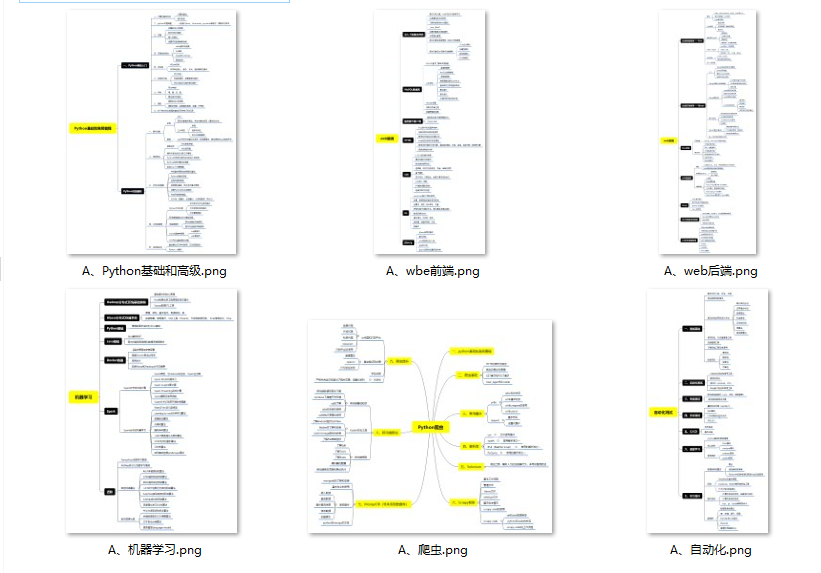### 二、Python必备开发工具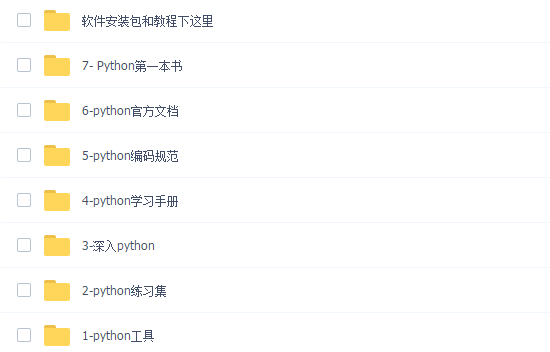### 三、精品Python学习书籍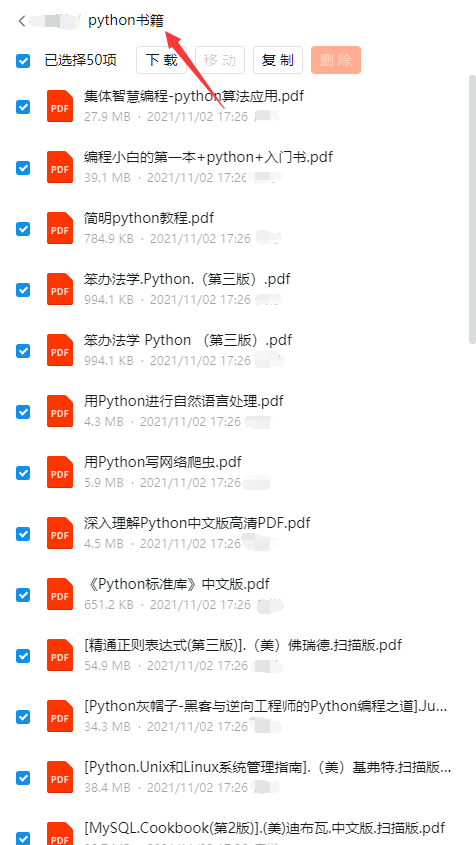### 四、Python视频合集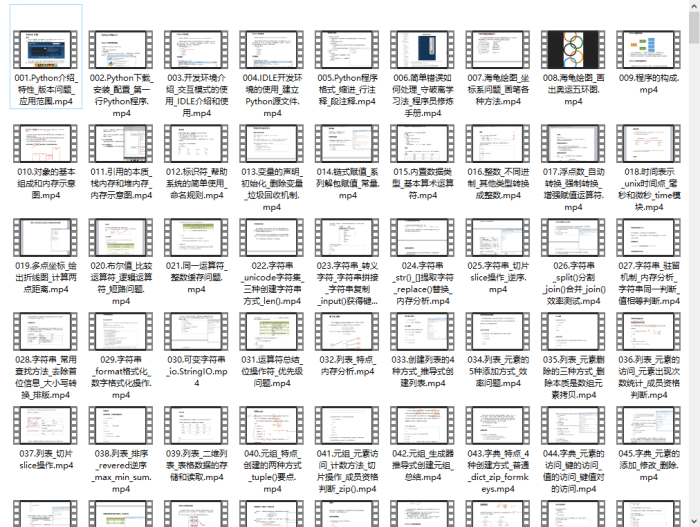### 五、实战案例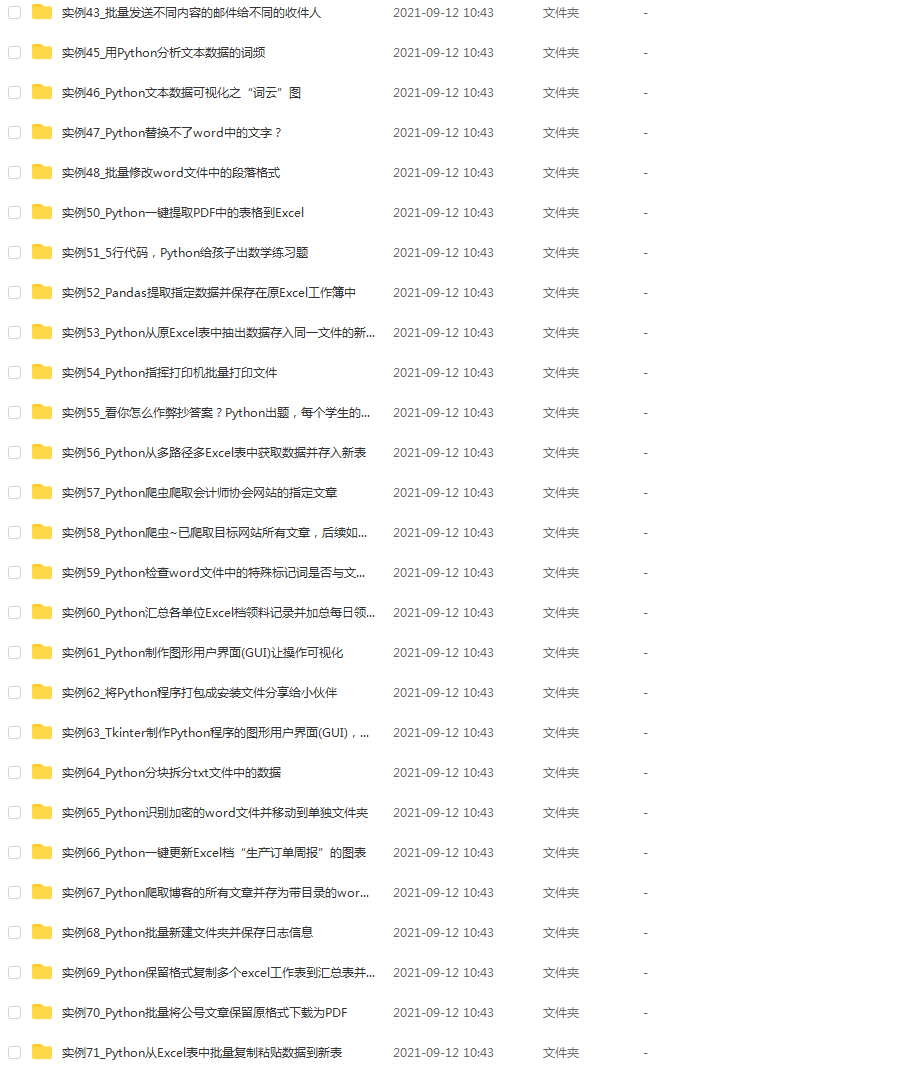### 六、Python练习题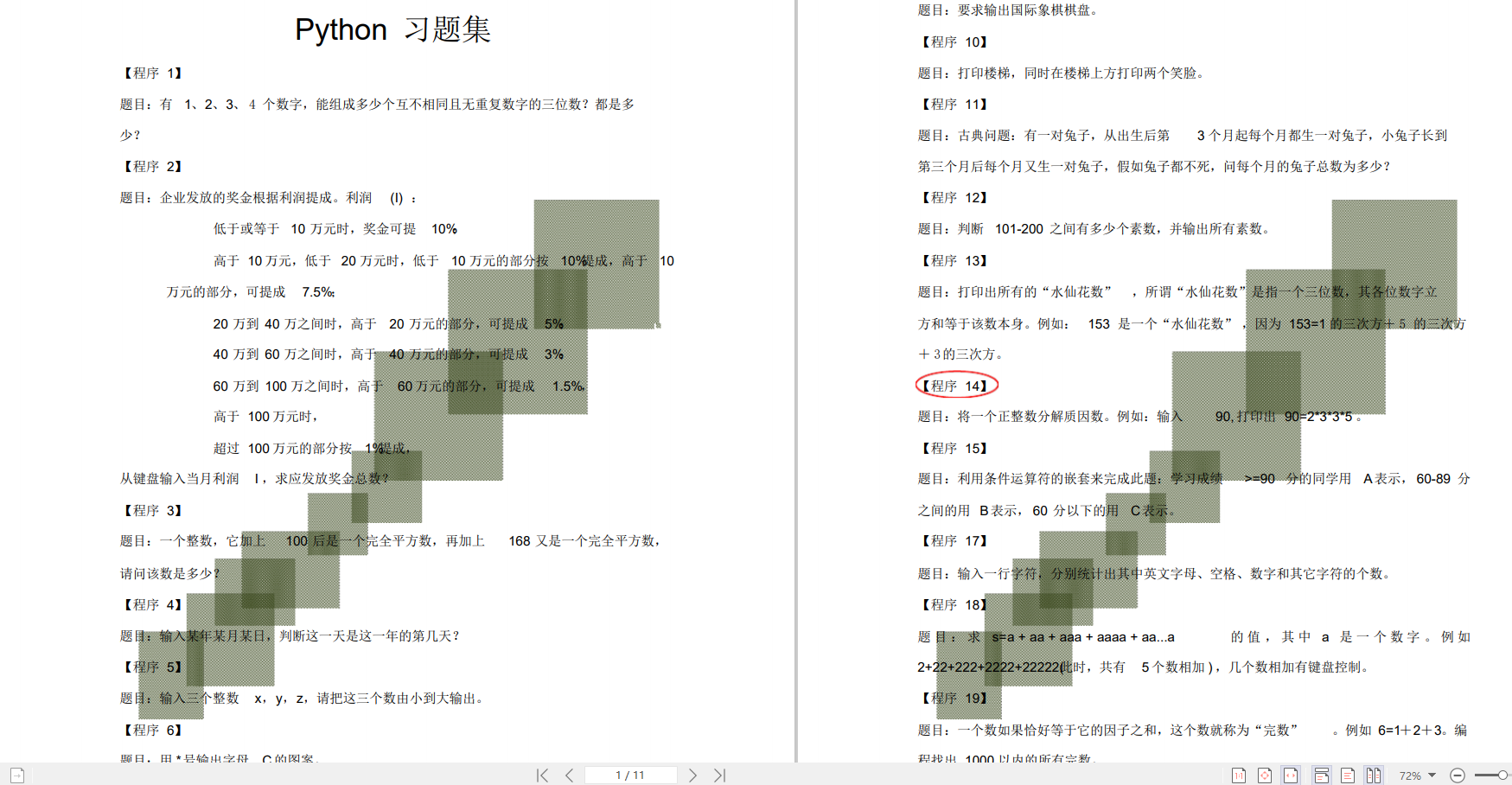### 七、面试资料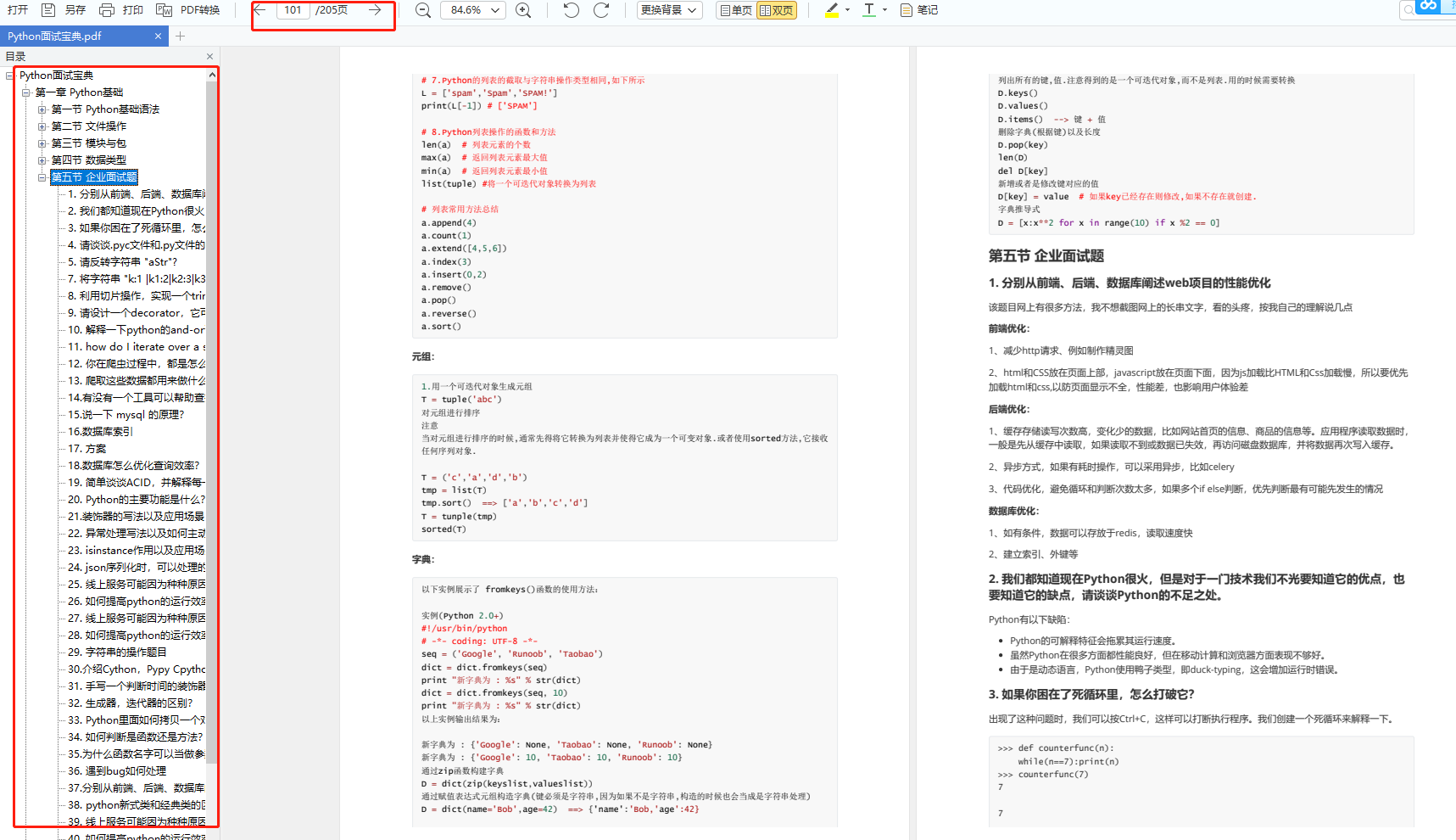##### 这份完整版的Python全套学习资料已经上传CSDN，朋友们如果需要可以微信扫描下方CSDN官方认证二维码免费领取【`保证100%免费`】